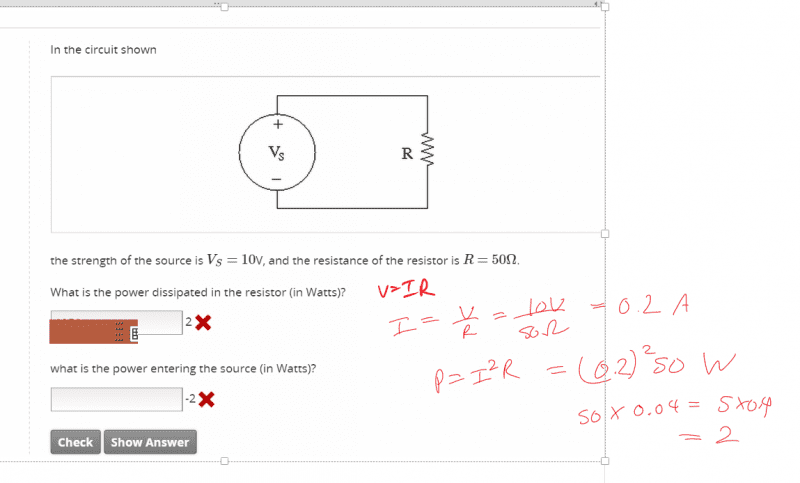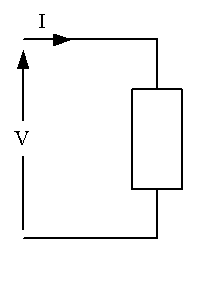# What does "power entering the source" mean?

• LongApple
In summary, the concept of power entering a source refers to the potential across the component multiplied by the current entering the component at the positive end of the potential. In the case of a voltage source with current coming out of the positive terminal, this is the opposite direction to the defined current in the figure. This idea is important in understanding the direction of current flow in circuits and can also lead to negative power dissipation in certain cases.

## Homework StatementV=IR
P=I^2 R[/B]

## The Attempt at a Solution

[/B]
What does it mean physically and intuitively "power entering the source" ?

That might be a start in getting me to understand why the answer is just negative the power dissipated in the resistance.

What does negative power entering the source mean physically and where does this negative come from mathematically in the equations?

#### Attachments

The power entering any two-terminal component is defined by the potential across the component multiplied by the current entering the component at the + end of the potential:In your case the component is a voltage source and the current is coming out of the + terminal. That's the opposite direction to the defined current in the figure...

This problem is getting you familiar with the idea that +ve and -ve currents imply a direction relative to some reference. The expression "power leaving a source is 2W" means the same as "power entering a source is -2W".

In case you are wondering it's quite reasonable for power to enter a source. Consider what happens when a battery is on charge. The battery still acts like a voltage source (even if its not a perfectly ideal voltage source).

Later on you will come across more complicated circuits where the direction of the current in part of the circuit isn't known or isn't obvious. To solve these circuits one approach is to arbitrarily define one direction as positive and solve the circuit equations. Then if the answer turns out to be negative it means the current is actually flowing the other way. It doesn't mean you got anything wrong.

gneill said:
The power entering any two-terminal component is defined by the potential across the component multiplied by the current entering the component at the + end of the potential:

View attachment 77715
In your case the component is a voltage source and the current is coming out of the + terminal. That's the opposite direction to the defined current in the figure...

What does the bolded mean? There is no direction arrow for the current in the diagram

Also a somewhat related question but can "power dissipated" ever have a negative sign?

Colloquially I would think not unless that circuit element is actually gaining energy in which case it is not really net dissipating power.

LongApple said:
Also a somewhat related question but can "power dissipated" ever have a negative sign?

Colloquially I would think not unless that circuit element is actually gaining energy in which case it is not really net dissipating power.
Since such quantities depend upon rigid definitions it's possible to have negative quantities under the right circumstances. For example, a voltage source pushing current into a circuit is exhibiting "negative power dissipation".

LongApple said:
What does the bolded mean? There is no direction arrow for the current in the diagram
? Do you not see the defined direction of the current ##I## in the figure? It's the arrow on the conductor.